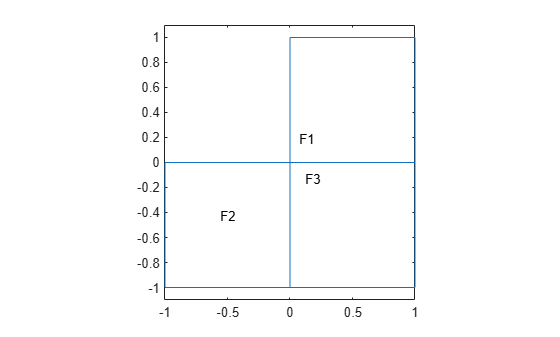findInitialConditions

Locate active initial conditions

Description

example

ic = findInitialConditions(ics,RegionType,RegionID) returns the active initial condition assignment ic for the initial conditions in the specified region.

Examples

collapse all

This example shows find the active initial conditions for a region.

Create a PDE model that has a few subdomains.

model = createpde();
geometryFromEdges(model,@lshapeg);
pdegplot(model,'FaceLabels','on')
ylim([-1.1,1.1])
axis equalSet initial conditions on each pair of regions.

setInitialConditions(model,12,'Face',[1,2]);
setInitialConditions(model,13,'Face',[1,3]);
setInitialConditions(model,23,'Face',[2,3]);

Check the initial conditions specification for region 1.

ics = model.InitialConditions;
ic = findInitialConditions(ics,'Face',1)
ic =
GeometricInitialConditions with properties:

RegionType: 'face'
RegionID: [1 3]
InitialValue: 13
InitialDerivative: []

Input Arguments

collapse all

Model initial conditions, specified as the InitialConditions property of a PDE model. Initial conditions can be complex numbers.

Example: model.InitialConditions

Geometric region type, specified as 'Edge' for a 2-D model, 'Face' for a 2-D model or 3-D model, or 'Cell' for a 3-D model.

Example: ca = findInitialConditions(ics,'Face',[1,3])

Data Types: char | string

Region ID, specified as a vector of positive integers. View the subdomain labels for a 2-D model using pdegplot(model,'FaceLabels','on'). Currently, there are no subdomains for 3-D models, so the only acceptable value for a 3-D model is 1.

Example: ca = findInitialConditions(ics,'Face',[1,3])

Data Types: double

Output Arguments

collapse all

Initial condition assignment, returned as a GeometricInitialConditions Properties or NodalInitialConditions Properties object.

Introduced in R2016a

Partial Differential Equation Toolbox Documentation평가판 신청